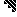# MINLPLib

### A Library of Mixed-Integer and Continuous Nonlinear Programming Instances

```Maximize determinant of 4 times 4 binary matrix
Let a(n) be the maximal determinant of a 0/1-matrix of size
n by n. Hadamard proved that a(n) ≤
attains this bound. The Hadamard conjecture states that this is the
case if and only if n+1 is 1 or 2 or a multiple of 4. The
values of a(n) for small n are known. See the on-line encyclopedia of integer
 Formatsⓘ ams gms mod nl osil pip Primal Bounds (infeas ≤ 1e-08)ⓘ 3.00000000 p1 ( gdx sol ) (infeas: 0) Other points (infeas > 1e-08)ⓘ Dual Boundsⓘ 3.00000000 (ANTIGONE)3.00000001 (BARON)3.00000000 (COUENNE)3.00000000 (LINDO)3.00000000 (SCIP) Sourceⓘ POLIP instance hadamard/hadamard_4 Applicationⓘ Linear Algebra Added to libraryⓘ 08 Dec 2018 Problem typeⓘ MBNLP #Variablesⓘ 17 #Binary Variablesⓘ 16 #Integer Variablesⓘ 0 #Nonlinear Variablesⓘ 16 #Nonlinear Binary Variablesⓘ 16 #Nonlinear Integer Variablesⓘ 0 Objective Senseⓘ max Objective typeⓘ linear Objective curvatureⓘ linear #Nonzeros in Objectiveⓘ 1 #Nonlinear Nonzeros in Objectiveⓘ 0 #Constraintsⓘ 1 #Linear Constraintsⓘ 0 #Quadratic Constraintsⓘ 0 #Polynomial Constraintsⓘ 1 #Signomial Constraintsⓘ 0 #General Nonlinear Constraintsⓘ 0 Operands in Gen. Nonlin. Functionsⓘ Constraints curvatureⓘ indefinite #Nonzeros in Jacobianⓘ 17 #Nonlinear Nonzeros in Jacobianⓘ 16 #Nonzeros in (Upper-Left) Hessian of Lagrangianⓘ 144 #Nonzeros in Diagonal of Hessian of Lagrangianⓘ 0 #Blocks in Hessian of Lagrangianⓘ 1 Minimal blocksize in Hessian of Lagrangianⓘ 16 Maximal blocksize in Hessian of Lagrangianⓘ 16 Average blocksize in Hessian of Lagrangianⓘ 16.0 #Semicontinuitiesⓘ 0 #Nonlinear Semicontinuitiesⓘ 0 #SOS type 1ⓘ 0 #SOS type 2ⓘ 0 Infeasibility of initial pointⓘ 0 Sparsity JacobianⓘSparsity Hessian of Lagrangianⓘ```\$offlisting
*
*  Equation counts
*      Total        E        G        L        N        X        C        B
*          1        0        1        0        0        0        0        0
*
*  Variable counts
*                   x        b        i      s1s      s2s       sc       si
*      Total     cont   binary  integer     sos1     sos2    scont     sint
*         17        1       16        0        0        0        0        0
*  FX      0
*
*  Nonzero counts
*      Total    const       NL      DLL
*         17        1       16        0
*
*  Solve m using MINLP maximizing objvar;

Variables  b1,b2,b3,b4,b5,b6,b7,b8,b9,b10,b11,b12,b13,b14,b15,b16,objvar;

Binary Variables  b1,b2,b3,b4,b5,b6,b7,b8,b9,b10,b11,b12,b13,b14,b15,b16;

Equations  e1;

e1.. b1*b6*b11*b16 - b1*b6*b12*b15 + b1*b8*b10*b15 - b4*b5*b10*b15 + b4*b5*b11*
b14 - b1*b8*b11*b14 + b1*b7*b12*b14 - b1*b7*b10*b16 + b3*b5*b10*b16 - b3*
b5*b12*b14 + b3*b8*b9*b14 - b4*b7*b9*b14 + b4*b7*b10*b13 - b3*b8*b10*b13
+ b3*b6*b12*b13 - b3*b6*b9*b16 + b2*b7*b9*b16 - b2*b7*b12*b13 + b2*b8*b11
*b13 - b4*b6*b11*b13 + b4*b6*b9*b15 - b2*b8*b9*b15 + b2*b5*b12*b15 - b2*b5
*b11*b16 - objvar =G= 0;

Model m / all /;

m.limrow=0; m.limcol=0;
m.tolproj=0.0;

\$if NOT '%gams.u1%' == '' \$include '%gams.u1%'

\$if not set MINLP \$set MINLP MINLP
Solve m using %MINLP% maximizing objvar;

```

Last updated: 2021-03-11 Git hash: 66559cbc